# How to use the LOOKUP function in Excel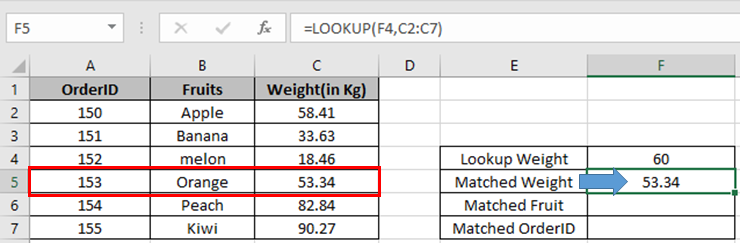In this article, we will learn How to use the LOOKUP function in Excel.

Why do we use the LOOKUP function ?

Whenever working with data tables. Sometimes we need to find the nearest approximate or exact match of the given value. For example, finding the employee having the salary growth around 40% or exactly 40%, if it exists. Let's learn the LOOKUP function Syntax and illustrate how to use the function in Excel below.

LOOKUP Function in Excel

LOOKUP function finds the estimated value in the range and returns the value from the range or corresponding value.

Syntax:

 =LOOKUP (lookup_value, lookup_vector, [result_vector])

lookup_value : value to look up for

lookup_vector : array where the function looks for the lookup_value.

[result vector] : [optional] if the return array is different from the lookup array.

Example :

All of these might be confusing to understand. Let's understand how to use the function using an example. Here we have some OrderID, Fruit name and respective weight bought by customers.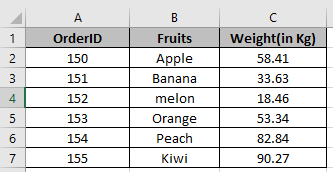There was a problem with an order where weight was around 60. Now we need to find the order where fruit weight was around 60. Use the LOOKUP formula to find the estimated value from the Weight column.

Formula:

 =LOOKUP(60, C2:C7)

60: lookup value

C2:C7: lookup range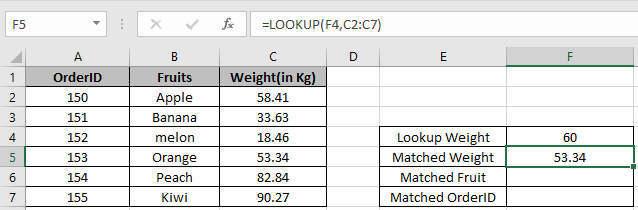As you can see the nearest weight in the cell.

Now we wish to find more details from Matched weight like fruit name

Use the formula to find the fruit name.

 =LOOKUP(F4,C2:C7,B2:B7)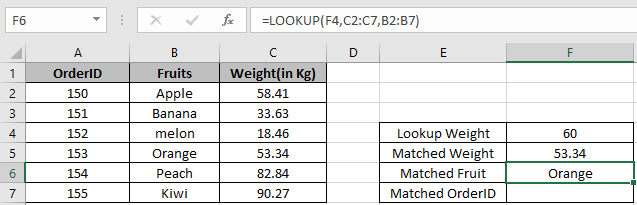As you can see the Fruit name corresponds to the nearest weight in the cell.

Use the formula to find the OrderID.

 =LOOKUP(F4,C2:C7,B2:B7)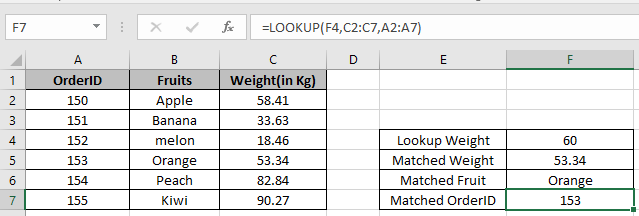As you can see the OrderID corresponds to the nearest weight in the cell.

You can also perform lookup exact matches using INDEX and MATCH function in Excel. Learn more about How to do Case Sensitive Lookup using INDEX & MATCH function in Excel. You can also look up for the partial matches using the wildcards in Excel.

Here are all the observational notes using the LOOKUP function in Excel
Notes :

1. Lookup array and matching array can be same or different
2. Sometimes the LOOKUP function returns the wrong value. So, you can use VLOOKUP or MATCH function

Hope this article about How to use the LOOKUP function in Excel is explanatory. Find more articles on finding values and related Excel formulas here. If you liked our blogs, share it with your friends on Facebook. And also you can follow us on Twitter and Facebook. We would love to hear from you, do let us know how we can improve, complement or innovate our work and make it better for you. Write to us at info@exceltip.com.

Related Articles :

How to use the MATCH function : MATCH function returns the matched index number matching the lookup value in the lookup array in Excel

Use INDEX and MATCH to Lookup Value : The INDEX & MATCH formula is used to lookup dynamically and precisely a value in a given table. This is an alternative to the VLOOKUP function and it overcomes the shortcomings of the VLOOKUP function.

Use VLOOKUP from Two or More Lookup Tables : To lookup from multiple tables we can take an IFERROR approach. To lookup from multiple tables, it takes the error as a switch for the next table. Another method can be an If approach.

How to do Case Sensitive Lookup in Excel : The excel's VLOOKUP function isn’t case sensitive and it will return the first matched value from the list. INDEX-MATCH is no exception but it can be modified to make it case sensitive.

Lookup Frequently Appearing Text with Criteria in Excel : The lookup most frequently appears in text in a range we use the INDEX-MATCH with MODE function.

Popular Articles :

How to use the IF Function in Excel : The IF statement in Excel checks the condition and returns a specific value if the condition is TRUE or returns another specific value if FALSE.

How to use the VLOOKUP Function in Excel : This is one of the most used and popular functions of excel that is used to lookup value from different ranges and sheets.

How to use the SUMIF Function in Excel : This is another dashboard essential function. This helps you sum up values on specific conditions.

How to use the COUNTIF Function in Excel : Count values with conditions using this amazing function. You don't need to filter your data to count specific values. Countif function is essential to prepare your dashboard.

Terms and Conditions of use

The applications/code on this site are distributed as is and without warranties or liability. In no event shall the owner of the copyrights, or the authors of the applications/code be liable for any loss of profit, any problems or any damage resulting from the use or evaluation of the applications/code.